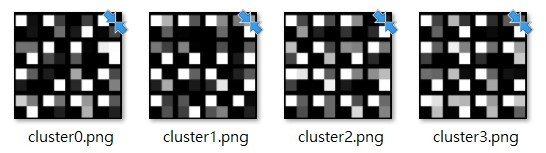DAY 7
0
AI & Data

# 前言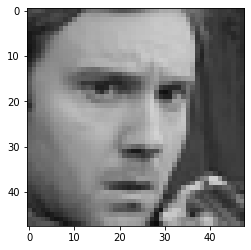# 如何決定最佳k數?

### 手肘法(Elbow Method)

SSE的程式碼實現:
`sum(np.min(cdist(X, X_mean, 'euclidean'), axis=1)) / sample_size)`

``````sample_size, nrows, ncols = X_features.shape
X = X_features.reshape((sample_size, nrows*ncols))
distortions = []
K = range(1, 10)
for k in K:
kmeans = KMeans(n_clusters=k)
kmeans.fit(X)
distortions.append(sum(np.min(cdist(X, kmeans.cluster_centers_, 'euclidean'), axis=1)) / sample_size)
``````

## 繪製手肘圖

``````plt.plot(K, distortions, 'bx-')
plt.xlabel('k')
plt.ylabel('Distortion')
plt.title('The Elbow Method showing the optimal k')
for k in range(1, 9):
plt.text(k+0.65, 0.3, f"{distortions[k]-distortions[k-1]:.2f}",
bbox=dict(facecolor='green', alpha=0.5))
plt.show()
``````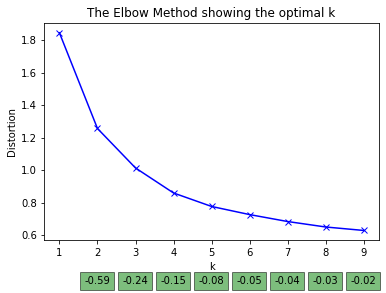# 結語

(雖然意義不好解釋，但這些特徵圖的確可以有效地讓神經網路分類器認識一張表情圖)

# 試試看:請嘗試描述各群特徵圖的含意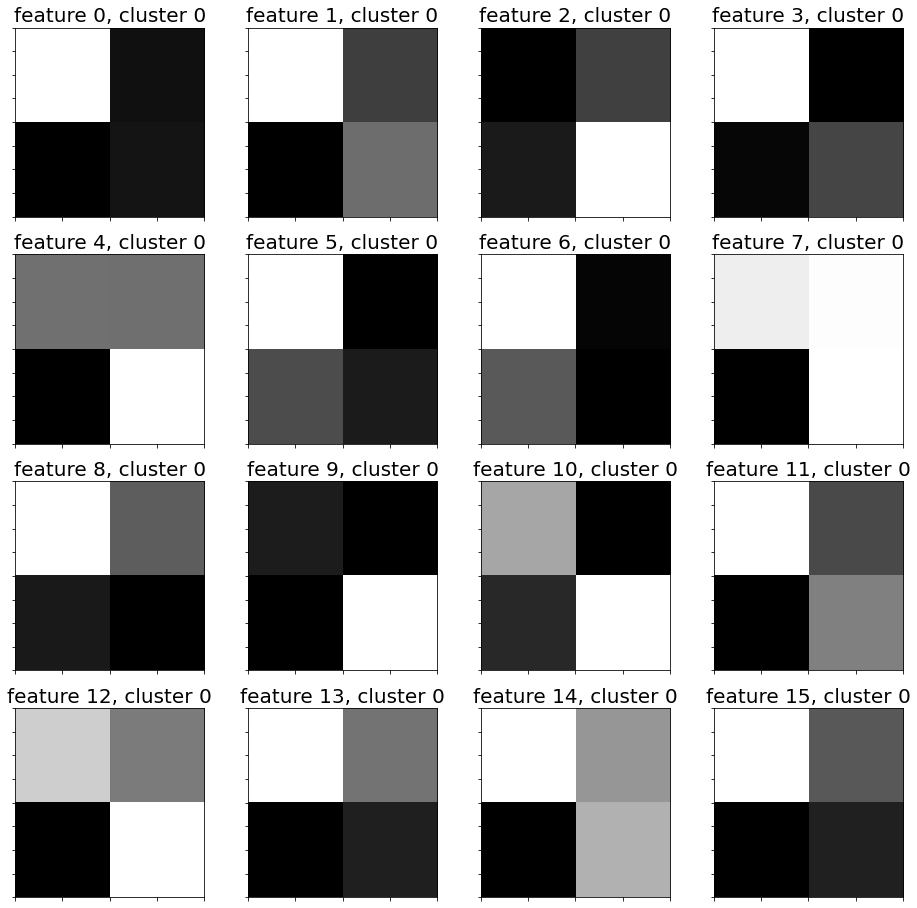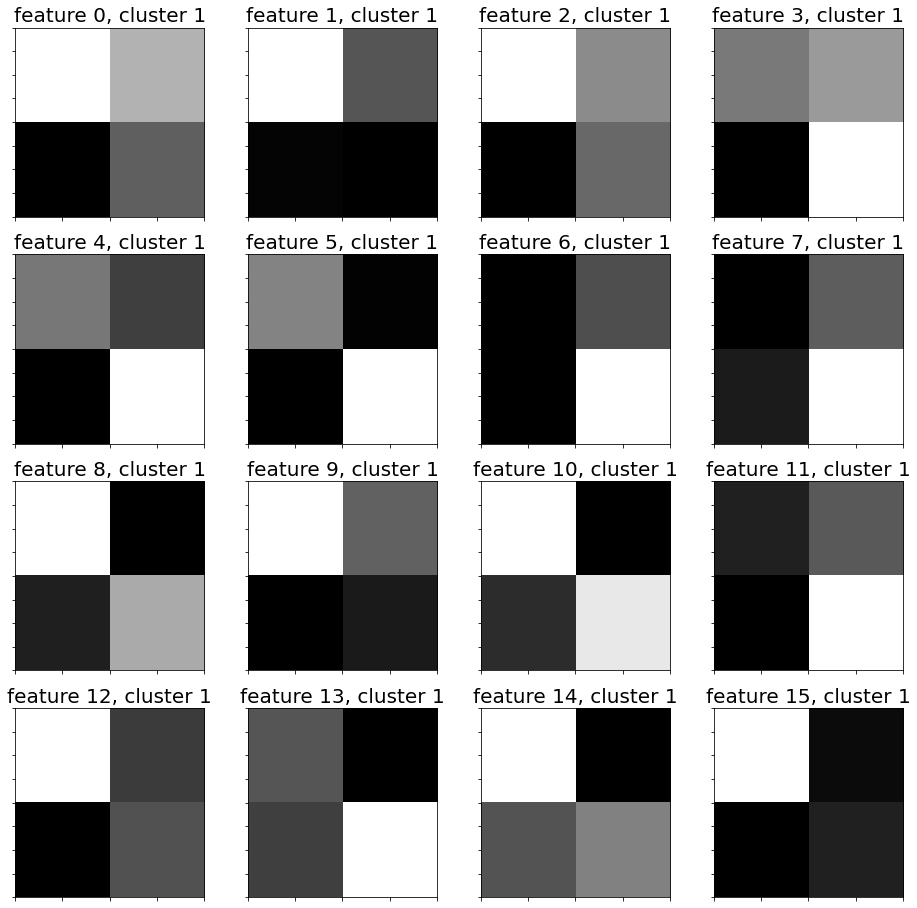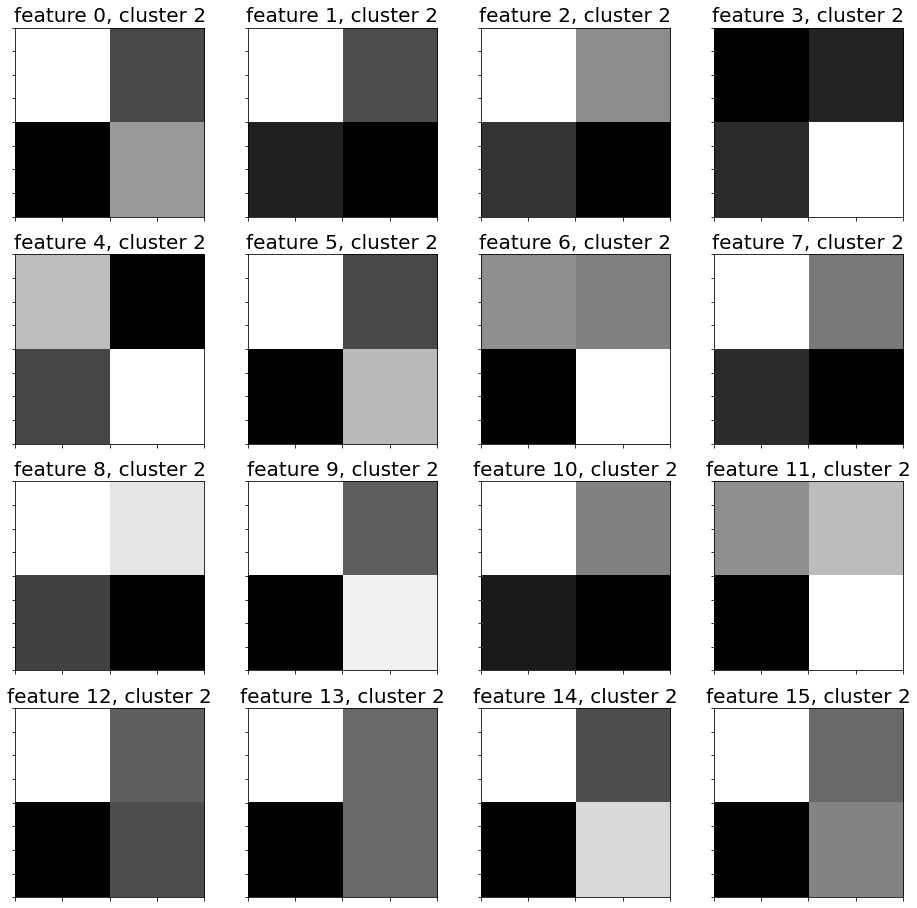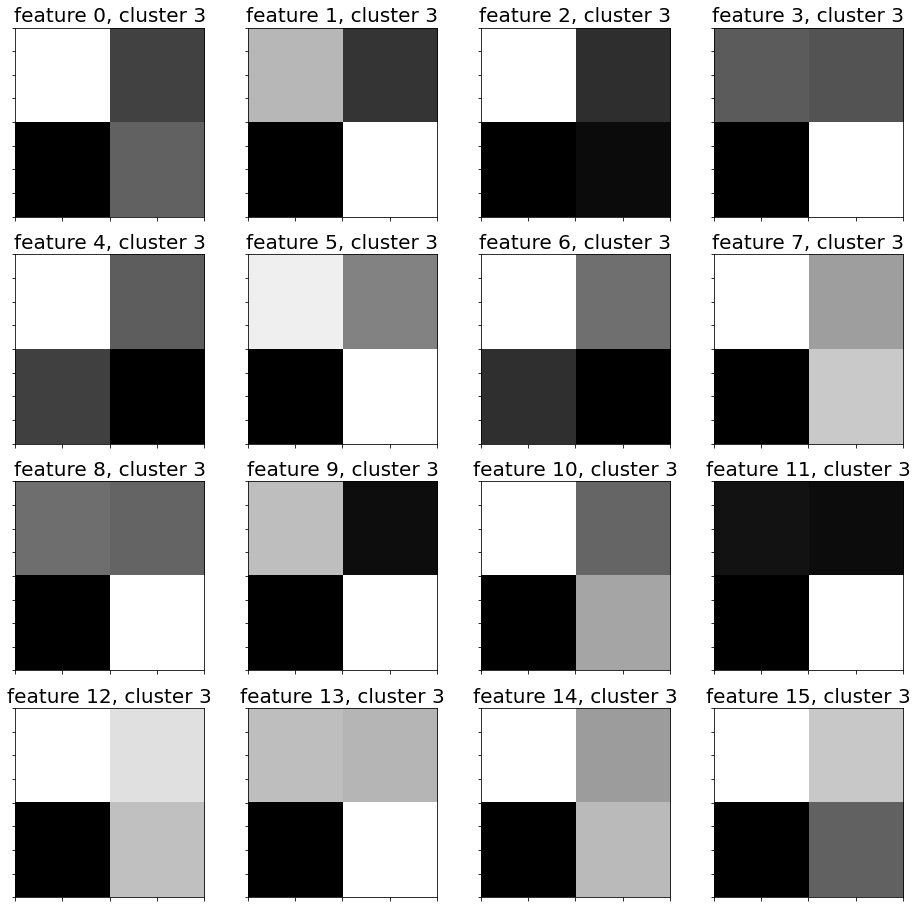## 縮小後合在一起看(反正我是看不出來XD